Lesson 44: Frequency, Wavelength, & Amplitude

Now that you know something about the properties of the two main types of waves (Lesson 43), we need to make sure that you can look at individual characteristics that waves can have.

• Not all waves are created equal!
• You need to be able to see the specific “faces” that each wave can have, based on three important characteristics: frequency, wavelength, and amplitude.

## Frequency

When we first started looking at SHM we defined period as the amount of time it takes for one cycle to complete... seconds per cycle

• Frequency is the same sort of idea, except we’re just going to flip things around.
• Frequency is a measurement of how many cycles can happen in a certain amount of time… cycles per second.
• If a motor is running so that it completes 50 revolutions in one second, I would say that it has a frequency of 50 Hertz.
• Hertz is the unit of frequency, and just means how many cycles per second.
• It is abbreviated as Hz.
• It is named after Heinrich Hertz, one member of the Hertz family that made many important contributions to physics.
• In formulas frequency appears as an "f".

Since frequency and period are exact inverses of each other, there is a very basic pair of formulas you can use to calculate one if you know the other…

It is very easy to do these calculations on calculators using the x-1 button.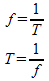Example 1: The period of a pendulum is 4.5s. Determine the frequency of this pendulum.

The period means that it will take 4.5 seconds for the pendulum to swing back and forth once. So, I expect that my frequency will be a decimal, since it will complete a fraction of a swing per second.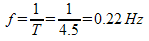## Wavelength

Wavelength is a property of a wave that most people (once they know what to look for) can spot quickly and easily, and use it as a way of telling waves apart. Look at the following diagram...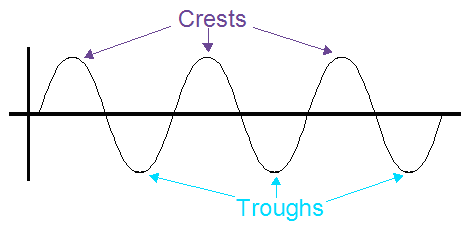Figure 1
• Any of the parts of the wave that are pointing up like mountains are called crests. Any part that is sloping down like a valley is a trough.
• Wavelength is defined as the distance from a particular height on the wave to the next spot on the wave where it is at the same height and going in the same direction.
• Usually it is measured in metres, just like any length.
• There isn’t a special spot you have to start on a wave to measure wavelength, just make sure you are back to the same height going in the same direction. Most people do like to measure from one crest to the next crest (or trough to trough), just because they are easy to spot.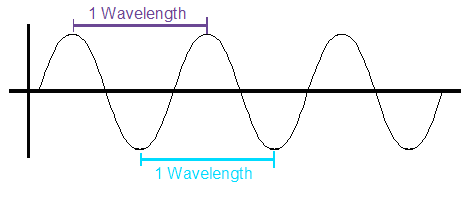Figure 2

On a longitudinal wave, the wavelength is measured as the distance between the middles of two compressions, or the middles of two expansions.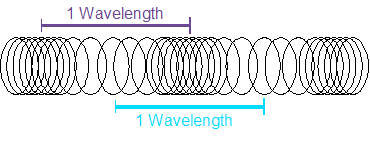Figure 3

This leads us to one of the most important formulas you will use when studying waves.

• Frequency tells us how many waves are passing a point per second, the inverse of time.
• Wavelength tells us the length of those waves in metres, almost like a displacement.
• If we multiply these two together, we are really multiplying 1/s and m… which gives us m/s, the velocity of the wave!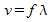v = velocity of the wave (m/s)
f = frequency (Hz)
λ = wavelength (m)

Example 2: A wave is measured to have a frequency of 60Hz. If its wavelength is 24cm, determine how fast it is moving.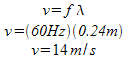Example 3: The speed of light is always 3.00e8 m/s. Determine the frequency of red light which has a wavelength of 700nm.

Be careful when changing the 700nm into metres. Some people get really caught up with changing it into regular scientific notation with only one digit before the decimal. Why bother? It's only being used in a calculation. You’ll probably just make a mistake changing the power of 10, so just substitute in the power for the prefix and leave everything else alone…700 nm = 700 x 10-9 m since “nano” is 10-9.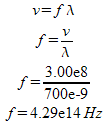## Amplitude

Amplitude is a measure of how big the wave is.

• Imagine a wave in the ocean. It could be a little ripple or a giant tsunami.
• What you are actually seeing are waves with different amplitudes.
• They might have the exact same frequency and wavelength, but the amplitudes of the waves can be very different.

The amplitude of a wave is measured as:

1. the height from the equilibrium point to the highest point of a crest or
2. the depth from the equilibrium point to the lowest point of a trough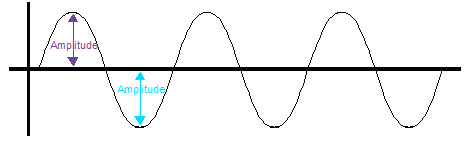Figure 4

When you measure the amplitude of a wave, you are really looking at the energy of the wave.

• It takes more energy to make a bigger amplitude wave.
• Anytime you need to remember this, just think of a home stereo’s amplifier… it makes the amplitude of the waves bigger by using more electrical energy.